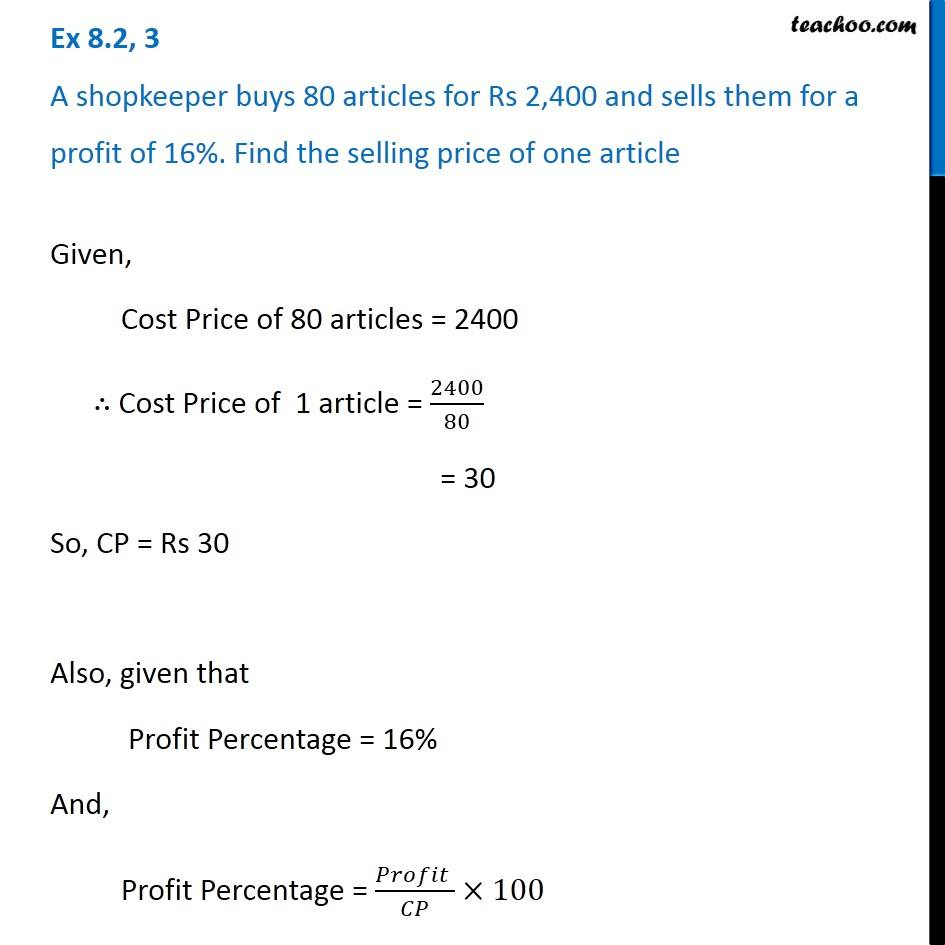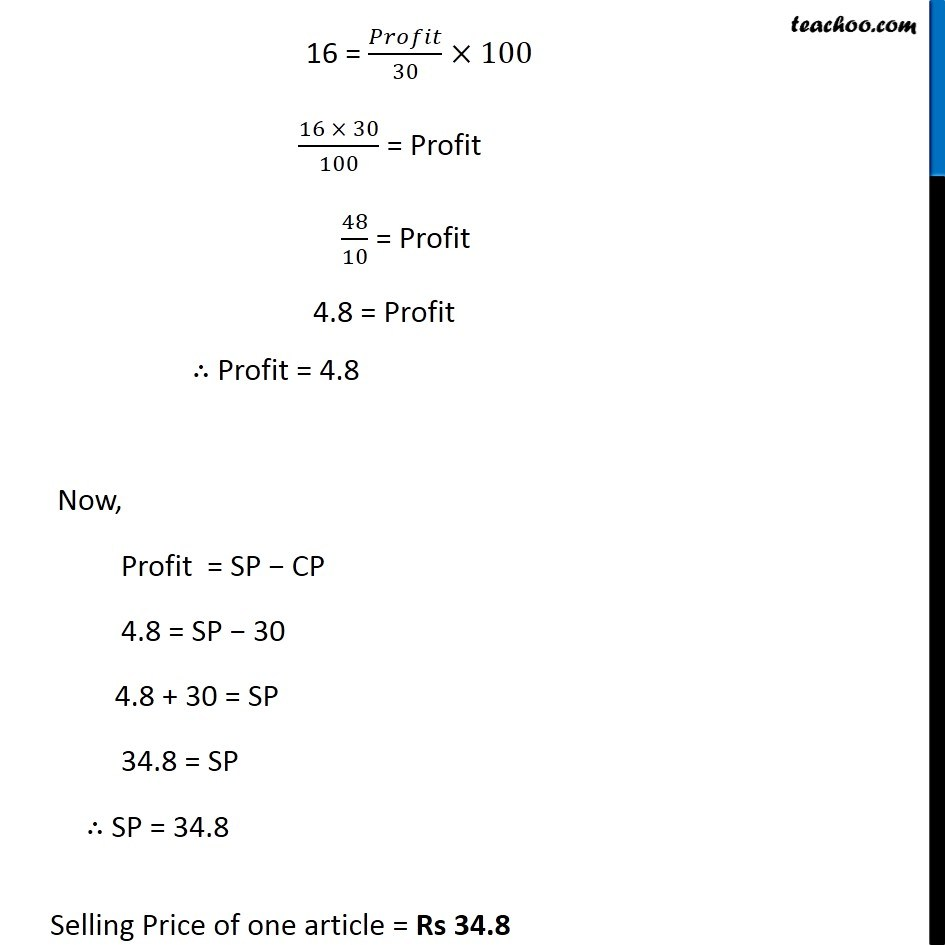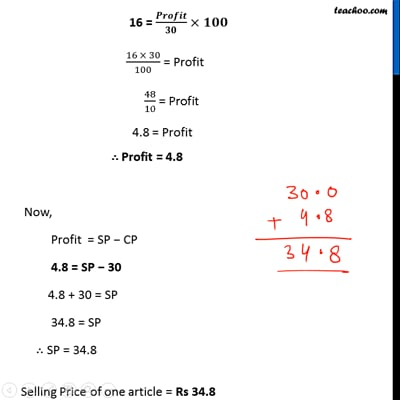Ex 7.2

Chapter 7 Class 8 Comparing Quantities
Serial order wiseThis video is only available for Teachoo black users

Learn in your speed, with individual attention - Teachoo Maths 1-on-1 Class

### Transcript

Question 3 A shopkeeper buys 80 articles for Rs 2,400 and sells them for a profit of 16%. Find the selling price of one article Given, Cost Price of 80 articles = 2400 ∴ Cost Price of 1 article = 2400/80 = 30 So, CP = Rs 30 Also, given that Profit Percentage = 16% And, Profit Percentage = (𝑃𝑟𝑜𝑓𝑖𝑡 )/𝐶𝑃×100 16 = 𝑃𝑟𝑜𝑓𝑖𝑡/30 ×100 (16 × 30)/100 = Profit 48/10 = Profit 4.8 = Profit ∴ Profit = 4.8 Now, Profit = SP − CP 4.8 = SP − 30 4.8 + 30 = SP 34.8 = SP ∴ SP = 34.8 Selling Price of one article = Rs 34.8